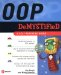# Multiple Inheritance Using C

## Multiple Inheritance Using C++

Previously in this chapter you learned that multiple inheritance is a way for a child to inherit from two unrelated parents. The example in this section shows how to implement multiple inheritance using C++.

This example introduces two new classes: Instructor and Worker . The Worker class contains attributes and methods for working with an income attribute. The Instructor class is derived from the Person class (inheriting the ID and the first and last name attributes and methods) as well as the Worker class. This is done to emphasize that a worker need not be a person to generate an income (for example, the worker could be a vending machine). However, an instructor is both a person and an income producer, so we use multiple inheritance.

The Instructor class has a parent-child relationship with both the Person class and the Worker class. The Person class and the Worker class are both base classes, and the Instructor class is the derived class.

Multiple inheritance is specified in the class header of the derived class, as shown in the Instructor class definition of this example. The class names of classes inherited by the derived class are specified to the right of the colon after the accessor specifier . Each class inherited by the Instructor class must be separated by a comma.

The result of this program is the same as the level inheritance example. The Write() member function of the Instructor class is passed information for all classes. The Display() member function is called to display those values on the screen.

Here is what is displayed on the screen when you run the following program:

ID: 102
First: Marcos
Last: Lopez
Income: 100000
Tenured: Yes

` #include <iostream>  #include <string.h>  using namespace std;  class Person  {  protected:  int m_ID;  char m_First, m_Last;  public:  Person()  {  m_ID = 0;  m_First = m_Last = '  #include < iostream >  #include <string.h>  using namespace std;  class Person  {  protected:  int m_ID;  char m_First, m_Last;  public:  Person()  {  m_ID = 0;  m_First = m_Last = '\0';  }  virtual void Display()  {  cout << "ID: " << m_ID << endl;  cout << "First: " << m_First << endl;  cout << "Last: " << m_Last << endl;  }  void Write( int ID, char First[], char Last[] )  {  m_ID = ID;  strcpy ( m_First, First );  strcpy( m_Last, Last );  }  };  class Worker  {  protected:  int m_Income;  public:  Worker()  {  m_Income = 0;  }  void Write( int Income )  {  m_Income = Income;  }  void Display()  {  cout << "Income: " << m_Income << endl;  }  };  class Instructor : public Person, public Worker  {  protected:  bool m_Tenured;  public:  Instructor()  {  m_Tenured = false;  }  void Write( int ID, char First[], char Last[], bool Tenured,  int Salary )  {  Person::Write( ID, First, Last );  Worker::Write( Salary );  m_Tenured = Tenured;  }  void Display()  {  Person::Display();  Worker::Display();  cout << "Tenured: " << (m_Tenured?"Yes":"No") << endl;  }  };  int main()  {  Instructor i;  i.Write( 102, "Marcos", "Lopez", true, 100000 );  i.Display();  return 0;  }  ';  }  virtual void Display()  {  cout << "ID: " << m_ID << endl;  cout << "First: " << m_First << endl;  cout << "Last: " << m_Last << endl;  }  void Write( int ID, char First[], char Last[] )  {  m_ID = ID;  strcpy( m_First, First );  strcpy( m_Last, Last );  }  };  class Worker  {  protected:  int m_Income;  public:  Worker()  {  m_Income = 0;  }  void Write( int Income )  {  m_Income = Income;  }  void Display()  {  cout << "Income: " << m_Income << endl;  }  };  class Instructor : public Person, public Worker  {  protected:  bool m_Tenured;  public:  Instructor()  {  m_Tenured = false;  }  void Write( int ID, char First[], char Last[], bool Tenured,  int Salary )  {  Person::Write( ID, First, Last );  Worker::Write( Salary );  m_Tenured = Tenured;  }  void Display()  {  Person::Display();  Worker::Display();  cout << "Tenured: " << (m_Tenured?"Yes":"No") << endl;  }  };  int main()  {  Instructor i;  i.Write( 102, "Marcos", "Lopez", true, 100000 );  i.Display();  return 0;  } `OOP Demystified
ISBN: 0072253630
EAN: 2147483647
Year: 2006
Pages: 130

Similar book on Amazon